Navigation Panel:(These buttons explained below)Question Corner and Discussion Area

# How to Draw a Circle on a Computer

I am looking for an algorithm to generate a circle/arc of a given radius R in assembly language. An important factor to note is that I am using 8085 based assembly language for this and it does not have Square Root or Trigonometric functions.
Here are two methods for drawing a circle when there is no access to trigonometric functions or square roots.

One approach is to approximate sine and cosine functions by means of a Taylor series expansion. Usually there is only a need to compute a few terms of the sum. The expansions for sine and cosine are as follows:Here x is in radians (1 radian =degrees). If you truncate the sum at the nth term, the error will be less than the n+1st term in the series. (This is true in general for an alternating series in which the absolute values of the terms are strictly decreasing.)

To make the error estimates even better, one can use trigonometric identities such as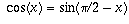and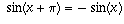to ensure that the angles used in these series are betweenand(the closer x is to 0, the fewer the number of terms which are needed to get the desired accuracy). For example, when x is in the range fromto, the expressionapproximates sin(x) with an error of at most 0.0025.

The other method is less mathematical and involves more brute force. Here a routine runs though all of the x and y values of the pixels on screen and sees if the point (x,y) lies on the circle. Actually, since the pixels are discrete, very few will actually lie on the mathematically ideal circle, but instead you can check if they are within a 1/2-pixel distance of the ideal circle by checking if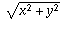is within 0.5 of R. This can be done without the square root function by seeing if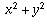lies between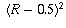(which equals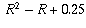) and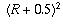(which equals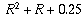).

This calculation can be performed using only integer calculations: for each pixel, evaluateand see if it is in the range fromto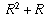inclusive. If so, colour the pixel on the screen, and if not, don't.

Although there are many more computations required for the second method, the calculations are much faster because they are integer calculations. This method would also make it much easier to colour in the interior of the circle or do other similar modifications to the output. The program could be further accelerated if there was some sort of a preliminary routine which decided which points were definitely not worth checking (for instance you would only need to check the points inside the smallest box containing the circle).

Which routine is faster may actually depend on the machine you are using. Some computers with math coprocessors handle decimal computations better than others.

[ Submit Your Own Question ] [ Create a Discussion Topic ]

This part of the site maintained by (No Current Maintainers)
Last updated: April 19, 1999
Original Web Site Creator / Mathematical Content Developer: Philip Spencer
Current Network Coordinator and Contact Person: Joel Chan - mathnet@math.toronto.edu

Navigation Panel: